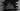# 3 ways in C# to find the sum and average of user input numbers## Different ways in C# to find the sum and average of user input numbers:

In this post, we will learn how to find the sum and average of n user input numbers. The program will take the numbers as inputs from the user and print the sum and average values.

We will learn different ways to find the sum and average values, by using a separate method, without a method, and by using a class.

### Method 1: C# program to find the sum and average of n user input numbers:

The below program reads n use input numbers and finds the sum and average of these numbers:

``````using System;

namespace Program
{
class Program
{
static void Main(string[] args)
{
int Total, Sum = 0, Value;
float Average;

Console.WriteLine("Enter the total count of numbers: ");

for (int i = 0; i < Total; i++)
{
Console.WriteLine("Enter a number: ");
Sum += Value;
}

Average = (float)Sum / Total;

Console.WriteLine("Sum: " + Sum + ", Average: " + Average);
}
}
}``````

Here,

• Total is an integer value to hold the total count of numbers. Sum is another integer to hold the sum of all user input numbers. Value is to hold the user input value. Average is a floating point variable to hold the average of the numbers.
• It takes the total count of numbers from the user as input and stores it in the variable Total.
• The for loop reads the numbers as inputs from the user and stores each value in the variable Value. Also, it adds those values to the Sum variable.
• Once the for loop ends, it finds the average value by dividing the sum by total number count. This value is converted to a floating point value and stores it in the Average variable.
• The last line is printing the calculated sum and average values.

If you run this program, it will print output as like below:

``````Enter the total count of numbers:
3
Enter a number:
1
Enter a number:
2
Enter a number:
4
Sum: 7, Average: 2.3333333

Enter the total count of numbers:
5
Enter a number:
1
Enter a number:
2
Enter a number:
3
Enter a number:
4
Enter a number:
5
Sum: 15, Average: 3``````

### Method 2: C# program to find the sum and average of n user input numbers by using a separate method:

We can also use a separate method to find the sum and average of n user input numbers. The program will take the total count as input from the user and call a separate method to find the sum and average.

``````using System;

namespace Program
{
class Program
{
static void findSumAverage(int Total)
{
float Average;
int Sum = 0, Value;

for (int i = 0; i < Total; i++)
{
Console.WriteLine("Enter a number: ");
Sum += Value;
}

Average = (float)Sum / Total;

Console.WriteLine("Sum: " + Sum + ", Average: " + Average);
}

static void Main(string[] args)
{
int Total;

Console.WriteLine("Enter the total count of numbers: ");

findSumAverage(Total);
}
}
}``````

Here,

• findSumAverage method finds the sum and average of Total number of user input numbers.
• We are using the same code as the previous example to find the sum and average values.

If you run this program, it will print similar output.

``````Enter the total count of numbers:
4
Enter a number:
2
Enter a number:
3
Enter a number:
4
Enter a number:
7
Sum: 16, Average: 4``````

### Method 3: C# program to find the sum and average of n user input numbers by using a separate class:

We can also use a class to find the sum and average of user input numbers. Let’s take a look at the below program:

``````using System;

namespace Program
{
class SumAverageCalculator
{
int Total, Sum = 0, Value;

{
Console.WriteLine("Enter the total count of numbers: ");

for (int i = 0; i < Total; i++)
{
Console.WriteLine("Enter a number: ");
Sum += Value;
}
}

public float GetAverage()
{
return (float)Sum / Total;
}

public int GetSum()
{
return Sum;
}
}
class Program
{
static void Main(string[] args)
{
SumAverageCalculator calculator = new SumAverageCalculator();

Console.WriteLine("Sum: " + calculator.GetSum() + ", Average: " + calculator.GetAverage());
}
}
}``````

Here,

• SumAverageCalculator is a separate class. This class has three public methods: ReadUserInput, GetAverage and GetSum.
• ReadUserInput method is used to read user input values, GetAverage is to get the average value and GetSum is to get the sum of all user input values.
• We are creating a new object of the class SumAverageCalculator and calling the ReadUserInput method first to read the user input numbers.
• The last line is calling GetSum() and GetAverage() methods on the object to find the sum and average values of the user input numbers.

If you run this program, it will print similar output.